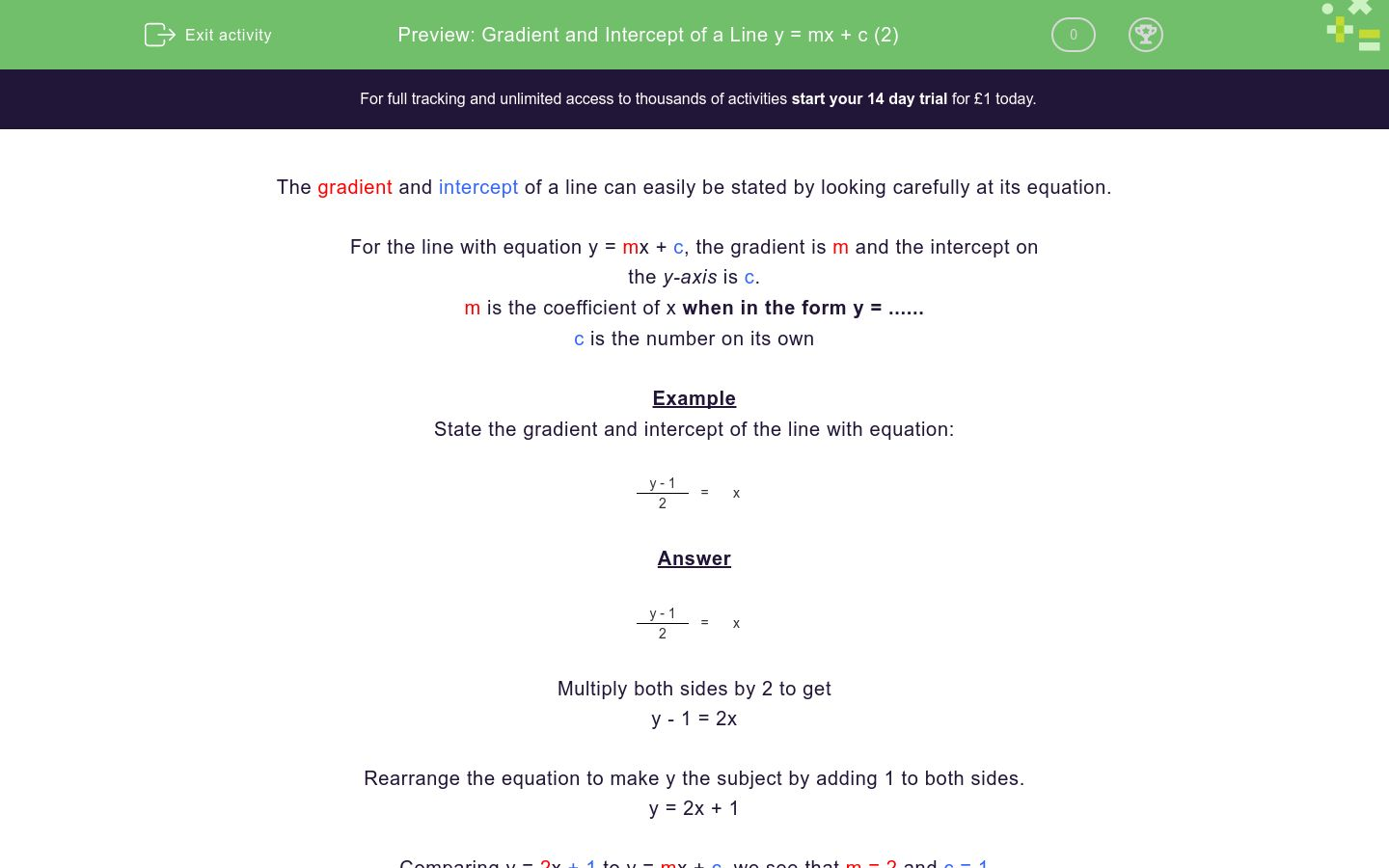# Gradient and Intercept of a Line y = mx + c (2)

In this worksheet, students state the gradient and intercept of a straight line given its equation.Key stage:  KS 4

Curriculum topic:  Algebra

Curriculum subtopic:  Use y = mx + c to Identify Parallel and Perpendicular Lines

Difficulty level:### QUESTION 1 of 10

The gradient and intercept of a line can easily be stated by looking carefully at its equation.

For the line with equation y = mx + c, the gradient is m and the intercept on

the y-axis is c.

m is the coefficient of x when in the form y = ......

c is the number on its own

Example

State the gradient and intercept of the line with equation:

 y - 1 = x 2

 y - 1 = x 2

Multiply both sides by 2 to get

y - 1 = 2x

Rearrange the equation to make y the subject by adding 1 to both sides.

y = 2x + 1

Comparing y = 2x + 1 to y = mx + c, we see that m = 2 and c = 1

The gradient of the line, m, is 2 and the intercept, c, is 1, which means that it crosses the y-axis at (0, 1)

Check

The line is shown below.

y = 2x + 1Note

The equation y = 2x + 1 can be rewritten in several ways as shown below.

They all represent the SAME line.

y = 1 + 2x

y - 1 = 2x

x = ½ (y - 1)

State the gradient of the line with equation:

 y - 3 = x 2

State the intercept on the y-axis of the line with equation:

 y - 4 = x 3

(3, 0)

(0, 3)

(4, 0)

(0, 4)

State the gradient of the line with equation:

y = 3 - 4x

State the gradient of the line with equation:

 y - 4 = x 2

State the gradient of the line with equation:

y = -7

0

x

7

-7

State the intercept on the y-axis of the line with equation:

 y + 6 = x 3

(-6, 3)

(0, -6)

(-6, 0)

(0, 3)

State the gradient of the line with equation:

 3 - y = x 2

State the gradient of the line with equation:

y = 4 - 0.3x

State the intercept on the y-axis of the line with equation:

 1 - y = x 4

(4, -1)

(0, 1)

(4, 0)

(0, -4)

State the intercept on the y-axis of the line with equation:

 y + 1 = x -2

(0, 2)

(0, -1)

(0, 1)

(2, -1)

• Question 1

State the gradient of the line with equation:

 y - 3 = x 2

2
• Question 2

State the intercept on the y-axis of the line with equation:

 y - 4 = x 3

(0, 4)
• Question 3

State the gradient of the line with equation:

y = 3 - 4x

-4
• Question 4

State the gradient of the line with equation:

 y - 4 = x 2

2
• Question 5

State the gradient of the line with equation:

y = -7

0
EDDIE SAYS
This is y = 0x - 7
• Question 6

State the intercept on the y-axis of the line with equation:

 y + 6 = x 3

(0, -6)
• Question 7

State the gradient of the line with equation:

 3 - y = x 2

-2
• Question 8

State the gradient of the line with equation:

y = 4 - 0.3x

-0.3
• Question 9

State the intercept on the y-axis of the line with equation:

 1 - y = x 4

(0, 1)
• Question 10

State the intercept on the y-axis of the line with equation:

 y + 1 = x -2